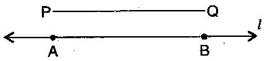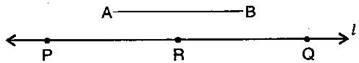### Practical Geometry-Solutions 14.3

CBSE Class –VI Mathematics
NCERT Solutions
Chaper 14 Practical Geometry (Ex. 14.3)

Question 1. Draw any line segment $\overline{\text{PQ}}.$ Without measuring $\overline{\text{PQ}},$ construct a copy of $\overline{\text{PQ}}.$(i) Given $\overline{\text{PQ}}$ whose length is not known.
(ii) Fix the compasses pointer on P and the pencil end on Q. The opening of the instrument now gives the length of $\overline{\text{PQ}}$.
(iii) Draw any line ${}^{\prime }{l}^{\prime }.$ Choose a point A on ${}^{\prime }{l}^{\prime }.$ Without changing the compasses setting, place the pointer on A.
Draw an arc that cuts ${}^{\prime }{l}^{\prime }$ at a point, say B. Now $\overline{\text{AB}}$ is a copy of $\overline{\text{PQ}}$.
Question 2. Given some line segment $\overline{\text{AB}},$ whose length you do not know, construct $\overline{\text{PQ}}$ such that the length of $\overline{\text{PQ}}$ is twice that of $\overline{\text{AB}}.$(i) Given $\overline{\text{AB}}$ whose length is not known.
(ii) Fix the compasses pointer on A and the pencil end on B. The opening of the instrument now gives the length of $\overline{\text{AB}}$.
(iii) Draw any line ${}^{\prime }{l}^{\prime }.$ Choose a point P on ${}^{\prime }{l}^{\prime }.$ Without changing the compasses setting, place the pointer on P.
(iv) Draw an arc that cuts ${}^{\prime }{l}^{\prime }$ at a point R.
(v) Now place the pointer on R and without changing the compasses setting, draw another arc that cuts ${}^{\prime }{l}^{\prime }$ at a point Q.
Thus $\overline{\text{PQ}}$ is the required line segment whose length is twice that of AB.